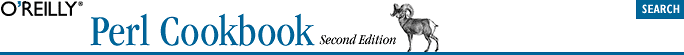home | O'Reilly's CD bookshelfs | FreeBSD | Linux | Cisco | Cisco Exam## 10.18. Program: Sorting Your Mail

The program in Example 10-1 sorts a mailbox by subject by reading input a paragraph at a time, looking for one with a "From" at the start of a line. When it finds one, it searches for the subject, strips it of any "Re: " marks, and stores its lowercased version in the @sub array. Meanwhile, the messages themselves are stored in a corresponding @msgs array. The \$msgno variable keeps track of the message number.

#### Example 10-1. bysub1

```  #!/usr/bin/perl
# bysub1 - simple sort by subject
my(@msgs, @sub);
my \$msgno = -1;
\$/ = '';                    # paragraph reads
while (<>) {
if (/^From/m) {
/^Subject:\s*(?:Re:\s*)*(.*)/mi;
\$sub[++\$msgno] = lc(\$1) || '';
}
\$msgs[\$msgno] .= \$_;
}
for my \$i (sort { \$sub[\$a] cmp \$sub[\$b] || \$a <=> \$b } (0 .. \$#msgs)) {
print \$msgs[\$i];
}```

That sort is only sorting array indices. If the subjects are the same, cmp returns 0, so the second part of the || is taken, which compares the message numbers in the order they originally appeared.

If sort were fed a list like (0,1,2,3), that list would get sorted into a different permutation, perhaps (2,1,3,0). We iterate across them with a for loop to print out each message.

Example 10-2 shows how an awk programmer might code this program, using the -00 switch to read paragraphs instead of lines.

#### Example 10-2. bysub2

```  #!/usr/bin/perl -n00
# bysub2 - awkish sort-by-subject
INIT { \$msgno = -1 }
\$sub[++\$msgno] = (/^Subject:\s*(?:Re:\s*)*(.*)/mi) if /^From/m;
\$msg[\$msgno] .= \$_;
END { print @msg[ sort { \$sub[\$a] cmp \$sub[\$b] || \$a <=> \$b } (0 .. \$#msg) ] }```

Example 10-3 is a program similar in spirit to Example 10-1 and Example 10-2.

#### Example 10-3. bysub3

```  #!/usr/bin/perl -00
# bysub3 - sort by subject using hash records
use strict;
my @msgs = ( );
while (<>) {
push @msgs, {
SUBJECT => /^Subject:\s*(?:Re:\s*)*(.*)/mi,
NUMBER  => scalar @msgs,   # which msgno this is
TEXT    => '',
} if /^From/m;
\$msgs[-1]{TEXT} .= \$_;
}

for my \$msg (sort {
\$a->{SUBJECT} cmp \$b->{SUBJECT}
||
\$a->{NUMBER}  <=> \$b->{NUMBER}
} @msgs
)
{
print \$msg->{TEXT};
}```

Once you have real hashes, adding further sorting criteria is simple. A common way to sort a folder is subject major, date minor order. The hard part is figuring out how to parse and compare dates. Date::Manip does this, returning a string you can compare; however, the datesort program in Example 10-4, which uses Date::Manip, runs more than 10 times slower than the previous one. Parsing dates in unpredictable formats is extremely slow.

#### Example 10-4. datesort

```  #!/usr/bin/perl -00
# datesort - sort mbox by subject then date
use strict;
use Date::Manip;
my @msgs = ( );
while (<>) {
next unless /^From/m;
my \$date = '';
if (/^Date:\s*(.*)/m) {
(\$date = \$1) =~ s/\s+\(.*//;  # library hates (MST)
\$date = ParseDate(\$date);
}
push @msgs, {
SUBJECT => /^Subject:\s*(?:Re:\s*)*(.*)/mi,
DATE    => \$date,
NUMBER  => scalar @msgs,
TEXT    => '',
};
} continue {
\$msgs[-1]{TEXT} .= \$_;
}

for my \$msg (sort {
\$a->{SUBJECT} cmp \$b->{SUBJECT}
||
\$a->{DATE}    cmp \$b->{DATE}
||
\$a->{NUMBER}  <=> \$b->{NUMBER}

} @msgs
)
{
print \$msg->{TEXT};
}```

Example 10-4 is written to draw attention to the continue block. When a loop's end is reached, either because it fell through to that point or got there from a next, the whole continue block is executed. It corresponds to the third portion of a three-part for loop, except that the continue block isn't restricted to an expression. It's a full block, with separate statements.